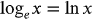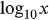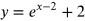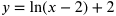1
155
views
34c
Problem

Problem 34c

Chapter 1: Functions and ModelsTextbook ExpertVerified Tutor
13 Oct 2021

Given information

Note that the given function is to draw two graphs of the natural logarithm function and the natural exponential each.

Since Natural logarithm;And common logarithm;is called the common logarithm.

Step-by-step explanation

Step 1.

Let us assume that,

1. Exponential function,2. Natural logarithm,